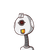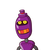# solve x – y = 1 & 2x + y = 8.... plz answer the question with correct answer mention the

solve x – y = 1 & 2x + y = 8
.
.
.
.
mention the method!!!! ​

### 2 thoughts on “solve x – y = 1 & 2x + y = 8<br />.<br />.<br />.<br />.<br /> plz answer the question with correct answer <br />mention the”

1.if x = 3

and y = 2

then 2x + y= 8

= 2 x 3 + 2 = 8

= 6 + 2 = 8

and therefore x – y = 1

3 – 2 = 1

2.## Solution ⬇️

### ✍️2x+y=8..(2)

_____________

### ️3x=9..(Adding equation 2 and 1)

_____________

$$= = > 3x = 9 \\ = = > x = \frac{9}{3} = 3$$

• Substituting value x=3 in equation 1

### ️ (x,y)(3,2) is the solution of the given quadratic equation

Step-by-step explanation:

hope you appreciate this ans